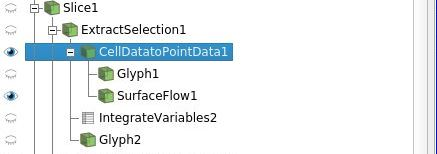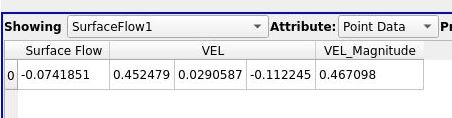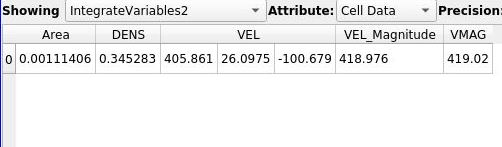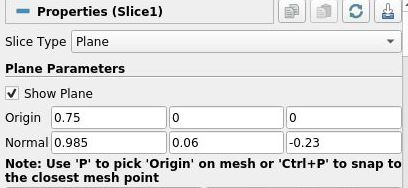# Calculating Mass Flow / Surface Flow

Hello,

i am trying to calculate the mass flow / surface flow through a slice inside a pipe, as you can see in this picture.

I know, there is a SurfaceFlow filter which should actually do this job for me, but i think i am using it wrong. To use this filter i need point data instead of cell data. And the filter gave me following results:But i dont get how paraview is calculating this SurfaceFlow value, because when i simply integrate all the variables of the cell data slice, i can multiplay VMAG, which is nearly perpendicular to the surface in this operating point, and the surface area.So this should give me nearly the same value as the SurfaceFlow filter, but it is totally different. Instead it is nearly the same value as VEL_Magnitude of SurfaceFlow1.
I really dont understand what im doing wrong. And why is the SurfaceFlow value negative? The velocity vector components have nearly the same direction as the surface normale of the slice.And to get the massflow i think i should use a calculator in the beginning multiplying the density and the velocity vector and then use this new vector for the SurfaceFlow filter, right?

I hope you can help me with this issue.
Thanks a lot!

Robin

In most cases, the SurfaceFlow value will be negative because the normal vectors of the cross section and the flow direction are opposite. To see the actual normal vectors of the cross section, you can use the Normal Glyphs filter.

If you need to align or reverse the normal vectors of a section, first apply the Extract Surface filter to convert data set to vtkPolyData. Then use the Generate Surface Normal filter to align the normals. Here, if you want to reverse the direction of the normals, turn on the Flip Normals option.

Also, if you are using a Multi-block DataSet, certain processes may fail. In such a case, using the Mege Block filter to merge the data into one before the various operations may improve the situation.

Hey,

the normal vector of the plane points in the direction of the flow, so it should definitely be positive, as you can see in the screenshots of the slice definition and the velocity components of the integrate variable filter.

But yes i am using a multi-block DataSet, but i extracted only one block using ExtractBlock before applying the following filters. Is this still a problem? Or could it be a problem using the Slice and ExtractSelection Filter?

I Found an alternative way to get the massflow and i think it works quite well.

1. Create a calculator to create the normalized normal vector of my slice plane

2. Calculate the “Massflow” for each Cell using the dot product of the Velocity Vector and Plane Normal Vector multiplying with the Density

3. Integrating the “Massflow” Variable and dont devide by the Area

But i still don’t understand what i am doing wrong with the SurfeFlow Filter…

The direction of the normal vector of the plane used in the Slice filter has nothing to do with the actual direction of the normal vector of the cross section. You may need to check the direction of the normal vector of the section with the Normal Glyphs filter.

Ok i see, but i don’t use the normal vectors of each cell. Instead i calculate my own general surface normal vector based on the normal direction of the slice definition and im actually pretty sure, that this must be correct. The results with this alternative method are valid, but the results with the SurfaceFlow Filter make 0 sense.

So is it neccesary for the SurfaceFlow Filter to align all the cellsurface normals in the same direction to work correctly?

Ok it is necessary… so now both methods deliver nearly the same results. But there is still an error of 2-3%. Could this be caused by the CellDataToPointData Filter? Because for the SurfaceFlow Filter i need PointData. With my alternative method i can stay with original CellData.